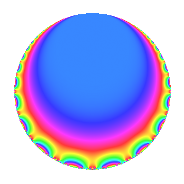# Properties

 Label 2880.1.j.aLevel 2880 Weight 1 Character orbit 2880.j Self dual yes Analytic conductor 1.437 Analytic rank 0 Dimension 1 Projective image $$D_{2}$$ CM/RM discs -4, -20, 5 Inner twists 4

# Related objects

## Newspace parameters

 Level: $$N$$ = $$2880 = 2^{6} \cdot 3^{2} \cdot 5$$ Weight: $$k$$ = $$1$$ Character orbit: $$[\chi]$$ = 2880.j (of order $$2$$, degree $$1$$, not minimal)

## Newform invariants

 Self dual: yes Analytic conductor: $$1.43730723638$$ Analytic rank: $$0$$ Dimension: $$1$$ Coefficient field: $$\mathbb{Q}$$ Coefficient ring: $$\mathbb{Z}$$ Coefficient ring index: $$1$$ Twist minimal: no (minimal twist has level 80) Projective image $$D_{2}$$ Projective field Galois closure of $$\Q(i, \sqrt{5})$$ Artin image $D_4$ Artin field Galois closure of 4.0.11520.1

## $q$-expansion

 $$f(q)$$ $$=$$ $$q - q^{5} + O(q^{10})$$ $$q - q^{5} + q^{25} + 2q^{29} + 2q^{41} - q^{49} + 2q^{61} - 2q^{89} + O(q^{100})$$

## Character values

We give the values of $$\chi$$ on generators for $$\left(\mathbb{Z}/2880\mathbb{Z}\right)^\times$$.

 $$n$$ $$577$$ $$641$$ $$901$$ $$2431$$ $$\chi(n)$$ $$-1$$ $$1$$ $$1$$ $$-1$$

## Embeddings

For each embedding $$\iota_m$$ of the coefficient field, the values $$\iota_m(a_n)$$ are shown below.

For more information on an embedded modular form you can click on its label.

Label $$\iota_m(\nu)$$ $$a_{2}$$ $$a_{3}$$ $$a_{4}$$ $$a_{5}$$ $$a_{6}$$ $$a_{7}$$ $$a_{8}$$ $$a_{9}$$ $$a_{10}$$
1279.1
 0
0 0 0 −1.00000 0 0 0 0 0
 $$n$$: e.g. 2-40 or 990-1000 Significant digits: Format: Complex embeddings Normalized embeddings Satake parameters Satake angles

## Inner twists

Char Parity Ord Mult Type
1.a even 1 1 trivial
4.b odd 2 1 CM by $$\Q(\sqrt{-1})$$
5.b even 2 1 RM by $$\Q(\sqrt{5})$$
20.d odd 2 1 CM by $$\Q(\sqrt{-5})$$

## Twists

By twisting character orbit
Char Parity Ord Mult Type Twist Min Dim
1.a even 1 1 trivial 2880.1.j.a 1
3.b odd 2 1 320.1.h.a 1
4.b odd 2 1 CM 2880.1.j.a 1
5.b even 2 1 RM 2880.1.j.a 1
8.b even 2 1 720.1.j.a 1
8.d odd 2 1 720.1.j.a 1
12.b even 2 1 320.1.h.a 1
15.d odd 2 1 320.1.h.a 1
15.e even 4 2 1600.1.b.a 1
20.d odd 2 1 CM 2880.1.j.a 1
24.f even 2 1 80.1.h.a 1
24.h odd 2 1 80.1.h.a 1
40.e odd 2 1 720.1.j.a 1
40.f even 2 1 720.1.j.a 1
40.i odd 4 2 3600.1.e.a 1
40.k even 4 2 3600.1.e.a 1
48.i odd 4 2 1280.1.e.a 2
48.k even 4 2 1280.1.e.a 2
60.h even 2 1 320.1.h.a 1
60.l odd 4 2 1600.1.b.a 1
120.i odd 2 1 80.1.h.a 1
120.m even 2 1 80.1.h.a 1
120.q odd 4 2 400.1.b.a 1
120.w even 4 2 400.1.b.a 1
168.e odd 2 1 3920.1.j.a 1
168.i even 2 1 3920.1.j.a 1
168.s odd 6 2 3920.1.bt.b 2
168.v even 6 2 3920.1.bt.b 2
168.ba even 6 2 3920.1.bt.a 2
168.be odd 6 2 3920.1.bt.a 2
240.t even 4 2 1280.1.e.a 2
240.bm odd 4 2 1280.1.e.a 2
840.b odd 2 1 3920.1.j.a 1
840.u even 2 1 3920.1.j.a 1
840.cb even 6 2 3920.1.bt.a 2
840.cg odd 6 2 3920.1.bt.b 2
840.ct odd 6 2 3920.1.bt.a 2
840.cv even 6 2 3920.1.bt.b 2

By twisted newform orbit
Twist Min Dim Char Parity Ord Mult Type
80.1.h.a 1 24.f even 2 1
80.1.h.a 1 24.h odd 2 1
80.1.h.a 1 120.i odd 2 1
80.1.h.a 1 120.m even 2 1
320.1.h.a 1 3.b odd 2 1
320.1.h.a 1 12.b even 2 1
320.1.h.a 1 15.d odd 2 1
320.1.h.a 1 60.h even 2 1
400.1.b.a 1 120.q odd 4 2
400.1.b.a 1 120.w even 4 2
720.1.j.a 1 8.b even 2 1
720.1.j.a 1 8.d odd 2 1
720.1.j.a 1 40.e odd 2 1
720.1.j.a 1 40.f even 2 1
1280.1.e.a 2 48.i odd 4 2
1280.1.e.a 2 48.k even 4 2
1280.1.e.a 2 240.t even 4 2
1280.1.e.a 2 240.bm odd 4 2
1600.1.b.a 1 15.e even 4 2
1600.1.b.a 1 60.l odd 4 2
2880.1.j.a 1 1.a even 1 1 trivial
2880.1.j.a 1 4.b odd 2 1 CM
2880.1.j.a 1 5.b even 2 1 RM
2880.1.j.a 1 20.d odd 2 1 CM
3600.1.e.a 1 40.i odd 4 2
3600.1.e.a 1 40.k even 4 2
3920.1.j.a 1 168.e odd 2 1
3920.1.j.a 1 168.i even 2 1
3920.1.j.a 1 840.b odd 2 1
3920.1.j.a 1 840.u even 2 1
3920.1.bt.a 2 168.ba even 6 2
3920.1.bt.a 2 168.be odd 6 2
3920.1.bt.a 2 840.cb even 6 2
3920.1.bt.a 2 840.ct odd 6 2
3920.1.bt.b 2 168.s odd 6 2
3920.1.bt.b 2 168.v even 6 2
3920.1.bt.b 2 840.cg odd 6 2
3920.1.bt.b 2 840.cv even 6 2

## Hecke kernels

This newform subspace can be constructed as the kernel of the linear operator $$T_{17}$$ acting on $$S_{1}^{\mathrm{new}}(2880, [\chi])$$.

## Hecke Characteristic Polynomials

$p$ $F_p(T)$
$2$ 
$3$ 
$5$ $$1 + T$$
$7$ $$1 + T^{2}$$
$11$ $$( 1 - T )( 1 + T )$$
$13$ $$( 1 - T )( 1 + T )$$
$17$ $$( 1 - T )( 1 + T )$$
$19$ $$( 1 - T )( 1 + T )$$
$23$ $$1 + T^{2}$$
$29$ $$( 1 - T )^{2}$$
$31$ $$( 1 - T )( 1 + T )$$
$37$ $$( 1 - T )( 1 + T )$$
$41$ $$( 1 - T )^{2}$$
$43$ $$1 + T^{2}$$
$47$ $$1 + T^{2}$$
$53$ $$( 1 - T )( 1 + T )$$
$59$ $$( 1 - T )( 1 + T )$$
$61$ $$( 1 - T )^{2}$$
$67$ $$1 + T^{2}$$
$71$ $$( 1 - T )( 1 + T )$$
$73$ $$( 1 - T )( 1 + T )$$
$79$ $$( 1 - T )( 1 + T )$$
$83$ $$1 + T^{2}$$
$89$ $$( 1 + T )^{2}$$
$97$ $$( 1 - T )( 1 + T )$$